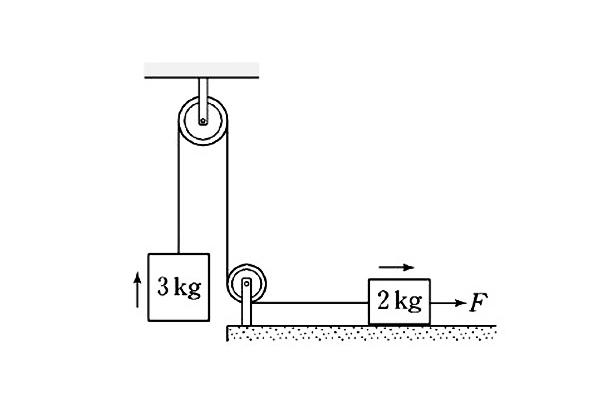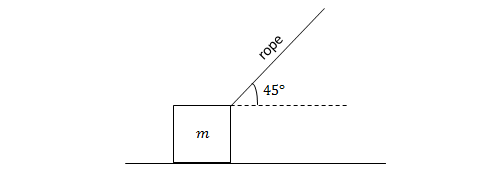Classical Mechanics

# FrictionWhen the force $F$ is acting toward the right on the 2 kg in the diagram, both objects are in uniform motion.

If the coefficient of kinetic friction between the 2 kg mass and the ground is $0.5,$ what is the magnitude of the force $F$ (in N)?

Gravitational acceleration is $g= 10 \text{ m/s}^2$.In the diagram above, a crate of mass $m=10\text{ kg}$ is being pulled across a horizontal floor by a rope at an angle of $45^\circ$ with the floor. The tension in the rope is $T=25\sqrt{2}\text{ N},$ and the coefficient of kinetic friction between the crate and the floor is $\mu=0.2.$ What is the acceleration of the crate?

The gravitational acceleration is $g=10\text{ m/s}^2.$

A coin of mass $3~\mbox{g}$ slides horizontally on the surface of a table. The frictional force exerted on the penny is $0.0147~\mbox{N}$. What is the coefficient of friction between the table and the penny?

Details and assumptions

• The acceleration of gravity is $-9.8~\mbox{m/s}^2$.

A very icy man who likes to travel decides that he wants to travel around the country in style. He buys a train to do his traveling on and gives it all sorts of neat cars - one car has a pool, one has a movie theatre, etc. His pride and joy is the ice rink car, a 30 meter long train car with the floor covered in ice so he can ice skate. Our icy guy is standing in the middle of his ice rink car, thinking what an icy guy he is, when his train begins to accelerate at $2~m/s^2$. How long does it take (in seconds) before our icy guy slides smack into the back of the train car?

Moral of the story: unlike laws made by people, the laws of physics apply equally to everyone, even people who love broom ball.

Details and assumptions

• The static and kinetic coefficients of friction between the ice guy's shoes and the ice is 0.1.
• The acceleration of gravity is $-9.8~m/s^2$.In the above diagram a force of $200$ N is pushing a brick weighing $50$ N against the wall, and the brick is static. If the coefficient of static friction is $0.5,$ what is the magnitude of static friction force on the brick (in N)?

×